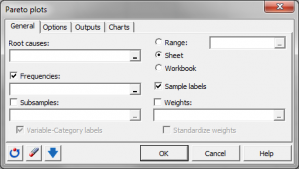# Pareto charts

Pareto charts allow to fastly evaluate differences in frequencies of levels in a qualitative variable. Available in Excel with the XLSTAT statistical software.## What is a Pareto chart

A Pareto plot displays both the frequency of occurrence, and the cumulative percentage of the total number of occurrences of level of a category variable. It draws its name from an Italian economist, but J. M. Juran is credited with being the first to apply it to industrial problems.

## Purpose of a Pareto plot

The purpose of the Pareto chart is to highlight the most important among a (typically large) set of factors. The causes that should be investigated (e. g., nonconforming items) are listed and percentages assigned to each one so that the total is 100 %. The percentages are then used to construct the diagram that is essentially a bar or pie chart. Pareto analysis uses the ranking of causes to determine which of them should be pursued first.

## Pareto chart in XLSTAT

XLSTAT offers you a large number of descriptive statistics and charts which give you a useful and relevant insight of your data, for a sample made up of N qualitative values:

• Number of observations
• Number of missing values
• Sum of weights*
• Mode*
• Frequency of mode*
• Category
• Frequency by category*
• Relative frequency by category*
• Cumulated relative frequency by category*

(*) Statistics followed by an asterisk take the weight of observations into account.

Although you can select several variables (or samples) at the same time, XLSTAT calculates all the descriptive statistics for each of the samples independently.

Several types of chart are available for qualitative data:

• Bar charts and Pie charts: Check this option to represent the frequencies or relative frequencies of the various categories of qualitative variables as bars or pieces of pie.
• Double pie charts: These charts are used to compare the frequencies or relative frequencies of sub-samples with those of the complete sample.
• Doughnuts and Stacked bars: these options are only checked if a column of sub-samples has been selected. These charts are used to compare the frequencies or relative frequencies of sub-samples with those of the complete sample.

Included in